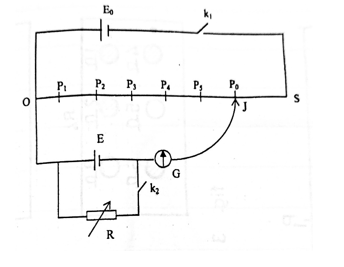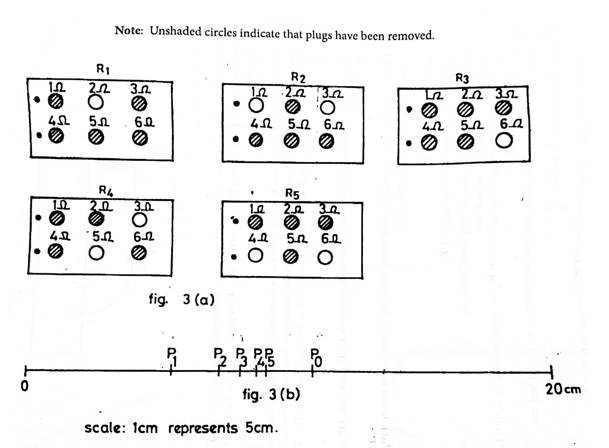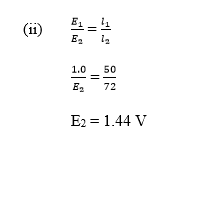Question 3In the circuit diagram above, OS is a uniform potentiometer wire of length 100 cm. Eo is an accumulator. E1 is a cell and R is a resistance box. J is a jockey and G is a centre-zero galvanometer.
With k1 closed while k2 is opened, a null-deflection is obtained at Po on OS.

This value OPo is measured and recorded. k2 is then closed and the resistance box is set at a known value R. The point of null-deflection P on OS is obtained and OP is measured and recorded. K2 is then opened.

The experiment is repeated four more increasing values of R. In each case, OP is measured and recorded.
Fig. 3(a) and fig. 3(b) illustrate the resistances Ri and the corresponding lengths OPi respectively, where i = 1, 2, 3, 4, and 5.
Fig 2(a) and Fig 2(b) illustrate the masses Mi and the corresponding lengths Li where i = 1. 2, 3, 4, 5, and 6.Read and record Ri.
Measure and record the raw lengths OPi.
Use the given scale to convert OPi to real lengths Xi.
Evaluate X-1 and R-1 in each case.
Plot a graph with X-1 on the vertical axis and R-1 on the horizontal axis, starting both axes from the origin (0,0).
Determine the slope, s, of the graph and the intercept, c, on the vertical axis.
Evaluate K1 = 1/c and K2 = s/c
State two precautions you would take if you would take if you were to perform this experiment in the laboratory.

(b) (i)State two advantages of using a potentiometer over a voltmeter for measuring potential difference.
(ii) A standard cell of emf 1.0 V is balanced against 50 cm length of a potentiometer wire. Calculate the emf of a cell that is balanced against 72 cm length of the same potentiometer wire.

Observation

Part (a) Candidates performance was below average.
Part (b) Candidates performance was below average.
The expected response is as stated below:

Po correctly measured and recorded to at least 1 d.p and within tolerance of ± 0.1 cm. Xo correctly converted

Five values of R correctly read and recorded in ohms (Deduct ½ mark for each wrong or missing value

Five values of OP correctly measured and record to at least 1 d.p and within tolerance of ± 0.1 cm. (Deduct ½ mark for each wrong or missing value)

Five values of X correctly converted. (Deduct ½ mark for each wrong or missing value)

Five values of X-1 correctly evaluated to at least 3 d.p. (Deduct ½ mark for each wrong or missing value)

Five values of R-1 correctly evaluated to at least 3 d.p. (Deduct ½ mark for each wrong or missing value Composite table showing at least R, OP, X, R-1 and X-1.

Plot a graph using reasonable scales draw line of best it

determine the slope and intercept of the graph
Evaluate K1 = 1/c
K2 = s/c

state any two of the following precautions.

e.g.
- Ensure tight connections
- Avoid parallax error when taking readings on metre rule/galvanometer
- Note/correct zero error on galvanometer
- Clean terminals